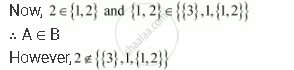# Determine Whether the Statement is True Or False. If It is True, Prove It. If It is False, Give an Example. If X Element a and a Element B, Then X Element B - Mathematics

True or False

Determine whether the statement is true or false. If it is true, prove it. If it is false, give an example.

If x ∈ A and A ∈ B, then x ∈ B

#### Solution

False

Let A = {1, 2} and B = {1, {1, 2}, {3}}Concept: Subsets
Is there an error in this question or solution?

#### APPEARS IN

NCERT Class 11 Mathematics
Chapter 1 Sets
Exercise 1.7 | Q 2.1 | Page 26